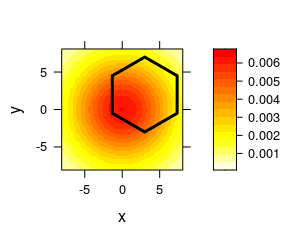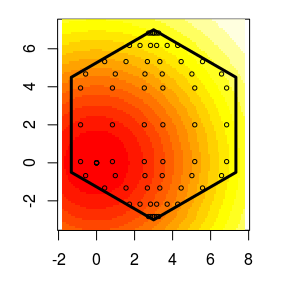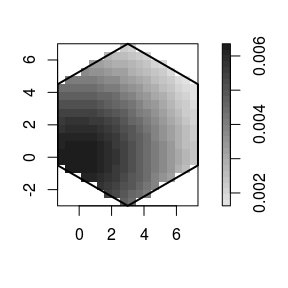# Getting started with polyCub

#### 2021-01-26The R package polyCub implements cubature (numerical integration) over polygonal domains. It solves the problem of integrating a continuously differentiable function f(x,y) over simple closed polygons.

For the special case of a rectangular domain along the axes, the package cubature is more appropriate (cf. CRAN Task View: Numerical Mathematics).

## Polygon representations

The integration domain is described by a polygonal boundary (or multiple polygons, including holes). Various R packages for spatial data analysis provide classes for polygons. The implementations differ in vertex order (which direction represents a hole) and if the first vertex is repeated.

All of polyCub’s cubature methods understand

• "owin" from package spatstat.geom,

• "gpc.poly" from rgeos (or gpclib),

• "SpatialPolygons" from package sp, and

• "(MULTI)POLYGON" from package sf.

Internally, polyCub uses its auxiliary xylist() function to extract a plain list of lists of vertex coordinates from these classes, such that vertices are ordered anticlockwise (for exterior boundaries) and the first vertex is not repeated (i.e., the "owin" convention).

## Cubature methods

The following cubature methods are implemented in polyCub:

1. polyCub.SV(): Product Gauss cubature

2. polyCub.midpoint(): Two-dimensional midpoint rule

3. polyCub.iso(): Adaptive cubature for radially symmetric functions $$f(x,y) = f_r(\lVert(x-x_0,y-y_0)\rVert)$$

4. polyCub.exact.Gauss(): Accurate (but slow) integration of the bivariate Gaussian density

The following section details and illustrates the different cubature methods.

## Illustrations

library("polyCub")

We consider the integration of a function f(x,y) which all of the above cubature methods can handle: an isotropic zero-mean Gaussian density. polyCub expects the integrand f to take a two-column coordinate matrix as its first argument (as opposed to separate arguments for the x and y coordinates), so:

f <- function (s, sigma = 5)
{
exp(-rowSums(s^2)/2/sigma^2) / (2*pi*sigma^2)
}

We use a simple hexagon as polygonal integration domain, here specified via an "xylist" of vertex coordinates:

hexagon <- list(
list(x = c(7.33, 7.33, 3, -1.33, -1.33, 3),
y = c(-0.5, 4.5, 7, 4.5, -0.5, -3))
)

An image of the function and the integration domain can be produced using polyCub’s rudimentary (but convenient) plotting utility:

plotpolyf(hexagon, f, xlim = c(-8,8), ylim = c(-8,8))### 1. Product Gauss cubature: polyCub.SV()

The polyCub package provides an R-interfaced C-translation of “polygauss: Matlab code for Gauss-like cubature over polygons” (Sommariva and Vianello, 2013, https://www.math.unipd.it/~alvise/software.html), an algorithm described in Sommariva and Vianello (2007, BIT Numerical Mathematics, https://doi.org/10.1007/s10543-007-0131-2). The cubature rule is based on Green’s integration formula and incorporates appropriately transformed weights and nodes of one-dimensional Gauss-Legendre quadrature in both dimensions, thus the name “product Gauss cubature”. It is exact for all bivariate polynomials if the number of cubature nodes is sufficiently large (depending on the degree of the polynomial).

For the above example, a reasonable approximation is already obtained with degree nGQ = 3 of the one-dimensional Gauss-Legendre quadrature:

polyCub.SV(hexagon, f, nGQ = 3, plot = TRUE)
#>  0.2741456The involved nodes (displayed in the figure above) and weights can be extracted by calling polyCub.SV() with f = NULL, e.g., to determine the number of nodes:

nrow(polyCub.SV(hexagon, f = NULL, nGQ = 3)[]$nodes) #>  72 For illustration, we create a variant of polyCub.SV(), which returns the number of function evaluations as an attribute: polyCub.SVn <- function (polyregion, f, ..., nGQ = 20) { nw <- polyCub.SV(polyregion, f = NULL, ..., nGQ = nGQ) ## nw is a list with one element per polygon of 'polyregion' res <- sapply(nw, function (x) c(result = sum(x$weights * f(x$nodes, ...)), nEval = nrow(x$nodes)))
structure(sum(res["result",]), nEval = sum(res["nEval",]))
}
polyCub.SVn(hexagon, f, nGQ = 3)
#>  0.2741456
#> attr(,"nEval")
#>  72

We can use this function to investigate how the accuracy of the approximation depends on the degree nGQ and the associated number of cubature nodes:

for (nGQ in c(1:5, 10, 20)) {
result <- polyCub.SVn(hexagon, f, nGQ = nGQ)
cat(sprintf("nGQ = %2i: %.12f (n=%i)\n", nGQ, result, attr(result, "nEval")))
}
#> nGQ =  1: 0.285265369245 (n=12)
#> nGQ =  2: 0.274027610314 (n=36)
#> nGQ =  3: 0.274145638288 (n=72)
#> nGQ =  4: 0.274144768964 (n=120)
#> nGQ =  5: 0.274144773834 (n=180)
#> nGQ = 10: 0.274144773813 (n=660)
#> nGQ = 20: 0.274144773813 (n=2520)

### 2. Two-dimensional midpoint rule: polyCub.midpoint()

The two-dimensional midpoint rule in polyCub is a simple wrapper around as.im.function() and integral.im() from package spatstat.geom. In other words, the polygon is represented by a binary pixel image and the integral is approximated as the sum of (pixel area * f(pixel midpoint)) over all pixels whose midpoint is part of the polygon.

To use polyCub.midpoint(), we need to convert our polygon to spatstat.geom’s “owin” class:

library("spatstat.geom")
hexagon.owin <- owin(poly = hexagon)

Using a pixel size of eps = 0.5 (here yielding 270 pixels), we obtain:

polyCub.midpoint(hexagon.owin, f, eps = 0.5, plot = TRUE)
#>  0.2736067### 3. Adaptive cubature for isotropic functions: polyCub.iso()

A radially symmetric function can be expressed in terms of the distance r from its point of symmetry: f(r). If the antiderivative of r times f(r), called intrfr(), is analytically available, Green’s theorem leads us to a cubature rule which only needs one-dimensional numerical integration. More specifically, intrfr() will be integrate()d along the edges of the polygon. The mathematical details are given in Meyer and Held (2014, The Annals of Applied Statistics, https://doi.org/10.1214/14-AOAS743, Supplement B, Section 2.4).

For the bivariate Gaussian density f defined above, the integral from 0 to R of r*f(r) is analytically available as:

intrfr <- function (R, sigma = 5)
{
(1 - exp(-R^2/2/sigma^2))/2/pi
}

With this information, we can apply the cubature rule as follows:

polyCub.iso(hexagon, intrfr = intrfr, center = c(0,0))
#>  0.2741448
#> attr(,"abs.error")
#>  3.043618e-15

Note that we do not even need the original function f.

If intrfr() is missing, it can be approximated numerically using integrate() for r*f(r) as well, but the overall integration will then be much less efficient than product Gauss cubature.

Package polyCub exposes a C-version of polyCub.iso() for use by other R packages (notably surveillance) via LinkingTo: polyCub and #include <polyCubAPI.h>. This requires the intrfr() function to be implemented in C as well. See https://github.com/bastistician/polyCub/blob/master/tests/polyiso_powerlaw.c for an example.

### 4. Integration of the bivariate Gaussian density: polyCub.exact.Gauss()

Abramowitz and Stegun (1972, Section 26.9, Example 9) offer a formula for the integral of the bivariate Gaussian density over a triangle with one vertex at the origin. This formula can be used after triangulation of the polygonal domain (polyCub currently uses tristrip() from the gpclib package). The core of the formula is an integral of the bivariate Gaussian density with zero mean, unit variance and some correlation over an infinite rectangle [h, Inf] x [0, Inf], which can be computed accurately using pmvnorm() from the mvtnorm package.

For the above example, we obtain:

gpclibPermit()  # accept gpclib license (prohibits commercial use)
#>  TRUE
polyCub.exact.Gauss(hexagon.owin, mean = c(0,0), Sigma = 5^2*diag(2))
#>  0.2741448
#> attr(,"nEval")
#>  48
#> attr(,"error")
#>  4.6e-14

The required triangulation as well as the numerous calls of pmvnorm() make this integration algorithm quiet cumbersome. For large-scale integration tasks, it is thus advisable to resort to the general-purpose product Gauss cubature rule polyCub.SV().

Note: polyCub provides an auxiliary function circleCub.Gauss() to calculate the integral of an isotropic Gaussian density over a circular domain (which requires nothing more than a single call of pchisq()).

## Benchmark

We use the last result from polyCub.exact.Gauss() as a reference value and tune the number of cubature nodes in polyCub.SV() and polyCub.midpoint() until the absolute error is below 10^-8. This leads to nGQ = 4 for product Gauss cubature and a 1200 x 1200 pixel image for the midpoint rule. For polyCub.iso(), we keep the default tolerance levels of integrate(). For comparison, we also run polyCub.iso() without the analytically derived intrfr function, which leads to a double-integrate approximation.

The median runtimes [ms] of the different cubature methods are given below.

benchmark <- microbenchmark::microbenchmark(
SV = polyCub.SV(hexagon.owin, f, nGQ = 4),
midpoint = polyCub.midpoint(hexagon.owin, f, dimyx = 1200),
iso = polyCub.iso(hexagon.owin, intrfr = intrfr, center = c(0,0)),
iso_double_approx = polyCub.iso(hexagon.owin, f, center = c(0,0)),
exact = polyCub.exact.Gauss(hexagon.owin, mean = c(0,0), Sigma = 5^2*diag(2)),
times = 6,
check = function (values) all(abs(unlist(values) - 0.274144773813434) < 1e-8))
summary(benchmark, unit = "ms")[c("expr", "median")]
expr median
SV 0.10
midpoint 243.81
iso 0.42
iso_double_approx 5.96
exact 8.93

The general-purpose SV-method is the clear winner of this small competition. A disadvantage of that method is that the number of cubature nodes needs to be tuned manually. This also holds for the midpoint rule, which is by far the slowest option. In contrast, the “iso”-method for radially symmetric functions is based on R’s integrate() function, which implements automatic tolerance levels. Furthermore, the “iso”-method can also be used with “spiky” integrands, such as a heavy-tailed power-law kernel $$f(r) = (r+1)^{-2}$$.